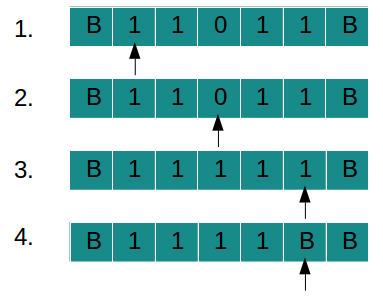1. Numbers are given in Uniary form
2. Example: 3 = 111, 2 = 11, 5 = 11111 etc.
3. For addition of 3 and 4, numbers will be given in TAPE as "B B 1 1 1 0 1 1 1 1 B B".
4. Convert '0' to '1' and reduce '1' from right
5. Hence output will be "B B 1 1 1 1 1 1 1 B B B"
6. Total number of '1' in output is = 7 which is addition of 3 and 4

### TAPE movement for string "110111":### Explanation of TAPE movement

1. Input is given as "11011" (2 + 2)
2. Scan string from left to right
3. Pass all '1'SSS and convert '0' to '1'
4. Reach BLANK in right
5. Convert rightmost '1' to BLANK
Addition of 2 and 2 = 4 is written on TAPE
Input String : 11011
Output String : 1111

### State Transition Diagram

We have designed state transition diagram for adder as follows:
1. Start scanning string from left to right
2. Pass all '1's and keep moving right
3. Convert '0' to '1' and keep moving right
4. When BLANK(in right) is reached move one step left convert '1' to BLANK
5. Keep moving left after that to point start of string
6. Number of '1's is reduced because number of '1' was increased by one while converting '0' to '1'

7.Uniary addition is like string concatination.